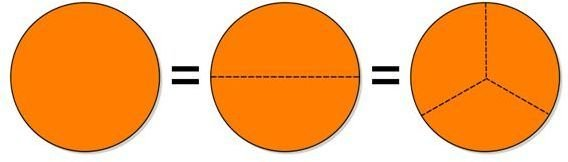# Comparing Fractions with Like Denominators: Information for Students

## Getting Ready to Compare Fractions

Comparing fractions can be tricky, especially when you have unlike denominators and improper fractions. Before you add, subtract, or compare fractions, you always want to make sure the denominators are the same; we'll cover how to do that next. Once we put them into the correct form, we'll discuss comparing fractions with like denominators.

Why do the denominators need to be the same? The denominator, or the bottom part of a fraction, is how many pieces something is divided into, while the numerator, or top part, is the number of those pieces you have. For example, suppose you're dividing up a pizza and taking half of it. You could just cut the pizza in half and take one piece, so you have 1/2 the pizza, or you could cut it into eight slices and take four of them, so you have 4/8 of the pizza. Either way, you get the same amount of pizza (including whatever gets stuck on the pizza cutter), and indeed 4/8 is the same as 1/2.

## Finding a Common Denominator

There are two ways to find a common denominator. One is simpler, while the other gives a better result.

Suppose the two fractions we want to compare are a/b and x/y, where b and y are different. One way to get a common denominator is to simply multiply both the top and bottom of the first fraction by y, and multiply both the top and bottom of the second fraction by b. This works because y/y = 1, and multiplying by 1 does not change the number.

This gives a/b * y/y = ay/by, and x/y * b/b = bx/by. We now have a common denominator! Going back to our pizza example, this would be like cutting each half of the first pizza into eight more slices, and cutting each slice of the second pizza in half; in each case, the pizza ends up with sixteen slices.

Of course, you generally don't want to have your pizza cut into 16 slices; they'll be awfully small! Generally, we will actually want to find the lowest common denominator. We can do this by using a factor tree to find the prime factors of each number, then multiply together all of the unique prime factors. In the example above, 2 = 2 and 8 = 2 * 2 * 2; the only prime factor is 2 and the most that is appears is three times, so the lowest common denominator is 23, or 8. Multiplying the first number by 4/4 and the second by 1/1 puts both numbers in terms of eights, so we can compare them.## Comparing Fractions with Like Denominators

Now that we have a common denominator, comparing the fractions is simple. Since the bottom number is the same, each item is divided into the same number of pieces, so we just need to compare how many pieces we have; in other words, the top number! In the example above, we found that 1/2 of a pizza was the same as 4/8 of a pizza, and 4/8 = 4/8, so 1/2 = 4/8; the two pizzas are the same.

Here are a few more comparisons; do you see why each one is true?

1/2 < 3/4

1/3 < 2/3

4/5 > 3/5